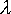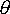QUESTION #316

# What is the wavelength of sodium light?

Sodium emits many lines in the visible region of the e.m. spectrum. Tabulations for the elements can be found in the Handbook of Chemistry and Physics [publ. Chemical Rubber Co.] but it is not a primary resource which can be found in monographs published by NIST.

The dominant yellow emissions you are probably referring to are the doublet located at: 588.9950 nm and 589.5924 nm having a relative intensity of about 2/1.
Answered by: Vince Calder, Ph.D., Physical Chemist, retired

The wavelength of Sodium light actually consists of two wavelengths called the D lines. They are:1 = 588.995 nm2 = 589.592 nm

These would easily be differentiated on a diffraction grating.

The angle of emergence, theta (), for a wavelength () is given by

d sin ()= n ()

So for each order n=1,2,... you will be able to see two lines very close to each other, the higher wavelength being the more diffracted. The zero order will just be seen as one line.

In the experiment for each order to the left of the zero order fringe1 would appear on the right and 2 on the left while for each order to the right of the zero fringe1 would appear on the left and2 on the right.